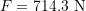### Problems: Chapter 8

1- A ladder, on which a person is standing, is leaning against the wall as shown. The floor has a coefficient of static friction as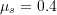, and the wall can be assumed frictionless (smooth) as it is covered by smooth tiles. The weight of the ladder acting at its center of gravity (the middle point of the length of the ladder) is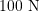. The weight of the person is the person is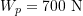and its location along the ladder is denoted asmeasured from point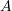shown in the figure. Determine how far the person can (slowly and with no acceleration) clime the ladder before the ladder slides. Hint: determineif the ladder is about to slide (impending motion).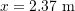2- With the constant assumptions of Problem 1, what should be the minimum inclination angle,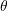of the ladder so that the person can reach and stand on the top of the ladder as shown?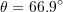Note that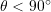3- Determine the minimum weight,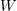, of the box on the horizontal surface so that the boxes stay stationary as shown.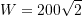4- The spool, shown in the figure, is holding a block weighing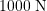. The rotation of the spool is controlled by a friction brake that can be activated if it is subjected to a pushing force,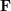as shown. If the coefficient of static friction at the contact surface of the spool and the brake is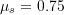, determine the minimum magnitude ofneeded to prevent the spool from rotation.